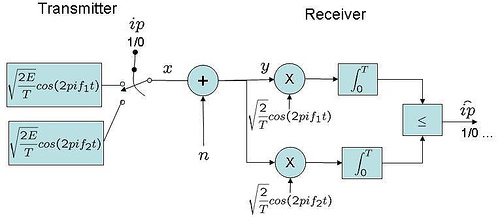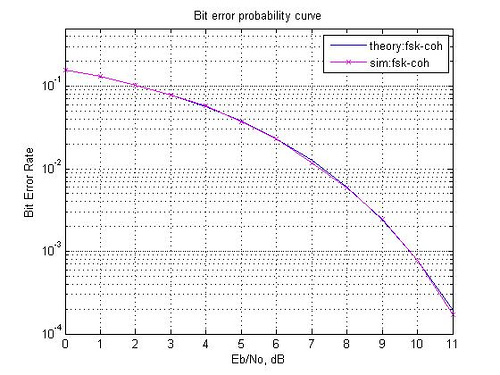- DSP log - http://www.dsplog.com -

Bit Error Rate (BER) for frequency shift keying with coherent demodulation

Posted By Krishna Sankar On August 30, 2007 @ 9:00 pm In Modulation | 22 Comments

Following the request by Siti Naimah , this post discuss the bit error probability for coherent demodulation of binary Frequency Shift Keying (BFSK) along with a small Matlab code snippet.

Using the definition provided in Sec 4.4.4 of [DIG-COMM-SKLAR]), in binary Frequency shift keying (BFSK), the bits 0′s and 1′s are represented by signals and having frequencies and respectively, i.e.

,

where

is the energy ,

is the symbol duration and

is an arbitrary phase (assume to be zero).

The two frequencies and are orthogonal, i.e.

and

.

Simple transmit-receive block diagram for binary frequency shift keying (FSK) can be as shown below.Figure: Block diagram of FSK modulation and coherent demodulation

For analyzing the bit error rate with coherent FSK demodulation, let us compare the signaling waveform used by binary FSK when compared with binary PSK . The distance between the energy of the signaling waveform for:

(a) binary phase shift keying (BPSK) is (uses antipodal signaling)

(b) binary frequency shift keying (BFSK) is (uses orthogonal signaling)

Figure: Orthogonal and antipodal signaling

Using similar mathematical formulation used for BPSK, but with the distance between the signals reduced by half, the bit error probability for coherent binary frequency shift keying is

. 

For obtaining the same bit error rate as BPSK, binary frequency shift keying requires around 3dB more . More details on the bit error curves with orthogonal and antipodal signals is discussed in Sec 3.2.5 of [DIG-COMM-SKLAR].

Simulation Model

Simple Matlab/Octave script for computing the bit error rate with FSK modulation. The code performs the following:

(a) Generation of random 1′s and 0′s

(b) Converting bits to appropriate frequency

(c) Passing through Additive White Gaussian Noise channel

(e) Counting the number of errors.Figure: Bit error probability with coherent demodulation of frequency shift keying

Hope this helps

Krishna

Reference

[DIG-COMM-SKLAR] Digital Communications: Fundamentals and Applications (2nd Edition), Bernard SklarURL to article: http://www.dsplog.com/2007/08/30/bit-error-rate-for-frequency-shift-keying-with-coherent-demodulation/

URLs in this post:

 request by Siti Naimah: http://www.dsplog.com/2007/08/05/bit-error-probability-for-bpsk-modulation/#comment-6